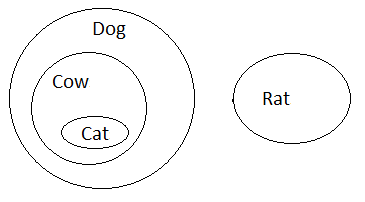Question of The Day30-06-2022

In the question below, there are three statements followed by two conclusions I and II. You have to take the given statements to be true even if they seem to be at variance from commonly known facts. Read the conclusions and then decide which of the given conclusions logically follows from the given statements disregarding commonly known facts.

Statements:

I: All cow are dog.

II: All cat are cow.

III: No rat is a dog.

Conclusions:

I: Atleast some cow are rat

II: No rat is a cat

Correct Answer : b ) Only conclusion II follows

Explanation :

Drawing a venn diagram using the given statementsChecking the Conclusion I,

Conclusion I: Atleast some cow are rat

According to statement I all cow are dog and according to statement III no rat is dog. Therefore, Conclusion I do not follows.

Checking the conclusion II,

Conclusion II: No rat is a cat

According to the given statements, no rat is dog and all cats are dog. Thus, conclusion II follows.

Therefore, only conclusion II follows.

Hence, (b) is the correct answer.0